## Welcome to the World of Modelling and Simulation

### What is Modelling?

This blog is all about system dynamics modelling and simulation applied in the engineering field, especially mechanical, electrical, and ...### Some Remarks on Forces at Contacts in Light of Bond Graph

So, you have already searched something related to "Contact Forces", and found this page. I welcome you to explore my perceptions behind the fundamental concept of contact forces which might satiate your query.

Figure 1 shows a single stage gear train. Torque T is applied on gear 1 which is further driving gear 2. The driver gear is the gear 1 and the driven gear is the gear 2. Here, I will try to explain the mechanics behind this gear mechanism. Figure 2 represents the torques and forces are acting on both of the gears. Gear 1 applies a force F1 which is the actual driving force to rotate the gear 2. This force F1 multiplied with the radius of the gear 2 generates eventually the driving torque T1. According to Newton’s third law, the force F1 applied by gear 1 on gear 2 will be equal and opposite to the force F2 which is the force applied by gear 2 on gear 1. The force F2 similarly generates a torque T2 on gear 1 by multiplication with gear 1 radius, but this torque is the resistive torque for gear 1 as the direction is opposite to the applied torque T. For two mating gears, their directions of angular displacements are opposite to one another, and if I am not wrong, this is physical in real life. For example, in figure 2, direction of angular displacement of gear 1 is clockwise whereas the direction of angular displacement of gear 2 is anti-clockwise.Figure 1: A single stage gear train.

Now, the question arises what is the type of the force F acting between the mating gears? Forces may be divided primarily as conservative and non-conservative forces. If it is conservative force, then the input and output energy must be balanced and the efficiency of the process is 100% which seems ideal. Now, if it is non-conservative force, then there would be energy dissipation from the system and work done by the non-conservative force on gear 2 is negative. Friction force is one of the common non-conservative forces that we experience in our daily life. My intuition in this regard is that this friction force cannot be the driving force for gear 2. The term “Driving Force”, by which, I mean the force helps to rotate gear 2. I will explain my point of view shortly, but before that let us have a look in figure 3 where the presence of the friction is considered. The nature of friction force is to oppose the motion of a moving body and in figure 3 this force acts opposite to the direction of rotation for both of the gears. In figure 3, there are also conservative forces (F1, F2) acting at the contact point p. What if there is only friction force acting at the contact? Then both of the gears would rotate in the same direction viz. clockwise in figure 3. But this is not physical in gear transmission. There have to be the presence of conservative forces with the friction as well.Figure 2: Free body diagram of the gear train system.

Now, I would like to explain the role of friction in both sliding and rolling motion. Figure 4 shows a sliding block on both horizontal and inclined planes. In figure 4, F is the applied force on the block which has mass M. While sliding on both the planes a frictional force f is acting between the block and the surface tries to resist the sliding motion of the block. And, the direction of friction is opposite to the direction of translational velocity of the block and it is true for every sliding motion.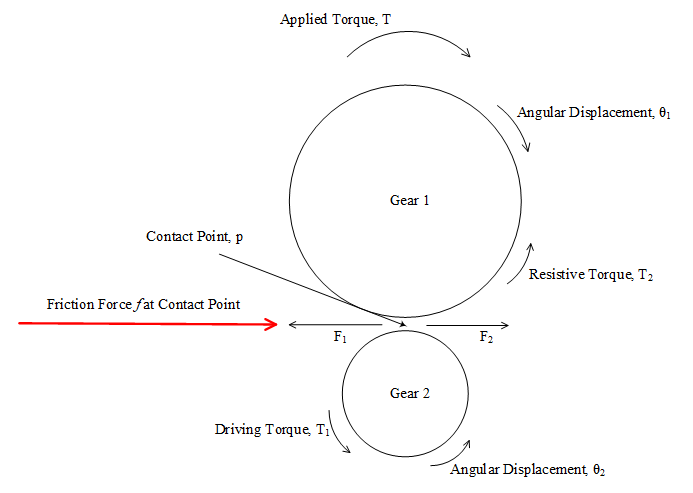Figure 3: Friction force acting at the contact point between gears.

The picture is different in case of rolling motion. Following figure 5, represents a ball rolling on horizontal plane as well as inclined plane. In this case, the friction force acts in the direction of the C.G. translational velocity of the ball. There is no sliding, and that is why, both the directions are same, however, this friction force tries to resist the rotation of the ball. And, eventually the friction force creates a torque which is acting opposite to the applied torque. Here, friction force helps to keep the rolling motion of the body and it is not the driving force. If it were the driving force, then the conservation of energy law would have been violated. Friction might be called driving force in a sense that it helps maintaining rolling motion of the wheel and if there were no friction then the wheel would have been sliding. We see the sliding occurs if a car tries to run over the black ice.

Figure 4: Sliding blocks on horizontal (a) and inclined (b) planes.

In previous figure 2, the force F is not the friction force, and the way, I considered this force is proportional to the relative tangential displacements of the mating gears. I added a constraint for this force and why I did this, will be enlightened in the next passages.

Figure 6 shows the bond graph model of the previous single stage gear train pictured in both figure 1 and 2. There is a special reason to show the bond graph model here although it could simply be done by applying Newton's law. In figure 6, the inertia of the gear 2 is derivative causal, and it is no more acting as a restoring element. It does not create a state of the system. Apparently, the model seems okay, but there is an inherent flaw in the model which does not make the model physical. The natural causality of inertial element is to take effort from the source to generate flow and the relationship is maintained by integration. But, if it is forced to acquire opposite causality, the relationship is governed by differentiation. To overcome this scenario, something has to be present to avoid this derivative causality. We say that the force F1 in figure 2 is the driving force for gear 2 but, how the force is generated? The answer to this question is due the contact between the mating gears.Figure 5: Rolling ball on horizontal (a) and inclined (b) planes.

So, gear 1 pushes gear 2 to rotate and according to Newton, gear 2 will try to resist and gives back reaction force. For this to be happened, there should have a medium to transfer the effort from gear 1 to gear 2. And, this medium may be modelled by a compliant element. It should be noted also that this compliant element does not represent contact deformation as it is not considered here. Although, in reality, there is eventually be microscopic deformation between mating gears, but for the case considered here this may be safely ignored. Figure 7 shows the compliant element C which integrates the tangential velocities of the mating gears and the force generated at the contact is proportional to the relative tangential displacements of the mating gears. The tangential velocity at the contact point is obtained by multiplying angular velocity with the radius of the gear and this is performed in the bond graph by TF elements.Figure 6: Bond graph model of the gear train in figure 2 (Derivative Causality).

Newton stated in his third law of motion that every action has an equal and opposite reaction. This is universally true and there is no doubt on this law. So, for an action on a body, according to this law there would be same and opposite reaction which means, Fa = -FrFigure 7: Bond graph model of the gear train in figure 2 (Integral Causality).

Where, Fa is the action force on a body and Fr is the reaction force by the body. But, the reaction force is created because of the contact or impact, and if we ignore the fact of force transmission between the contacting bodies we would have rigid mass coupling which again imposes derivative causality.

### Modelling a Multi-Degree of Freedom System with SIMULINK and Bond Graph

Differential equations of the system:

Notations:
m₁ = mass of the cart 1
m₂=mass of the cart 2
m₃=mass of the cart 3
m₄=mass of the cart 4

b₁=friction coefficient of cart 1
b₂=friction coefficient of cart 2
b₃=friction coefficient of cart 3
b₄=friction coefficient of cart 4

x₁=displacement of cart 1
x₂=displacement of cart 2
x₃=displacement of cart 3
x₄=displacement of cart 4

For Cart 1,

For Cart 2,

For Cart 3,

For Cart 4,

To solve the above differential equations two approaches are considered. At first the equations are simulated in SIMULINK and then validated by Bond Graph method .

Figure 1 represents the model of the mass-springs system. Four subsystems are used to show the differential equations of each mass. Figures 2, 3, 4 and 5 highlight the dynamic model for the cart 1, 2, 3 and 4 respectively.Figure 1: Showing the dynamic model of cart-springs system in SIMULINK.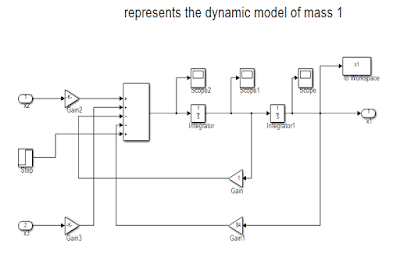Figure 2: Showing the dynamic model of cart 1.Figure 3: Showing the dynamic model of cart 2.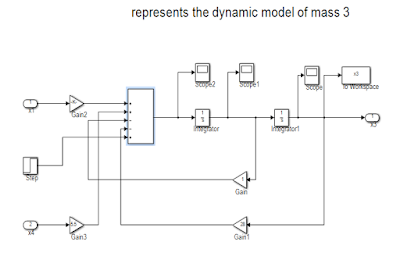Figure 4: Showing the dynamic model of cart 3.

Figure 5: Showing the dynamic model of cart 4.

Now, the parameters given in the question are used to get the simulation results. The simulated results show the position of the each cart with respect to time. Following figures 6, 7, 8, and 9 depict the position of the cart 1, 2, 3, and 4 respectively.

Figure 6: Showing the displacement of cart 1.

Figure 7: Showing the displacement of cart 2.

Figure 8: Showing the displacement of cart 3.

Figure 9: Showing the displacement of cart 4.

As it is mentioned earlier that to validate the model another method is also taken into consideration. The system is again simulated by bond graph technique. Bond graph depicts the picture of energy flow throughout the system and it considers the states which store energy which is eventually integrally causal element in the bond graph. Inertial (-I) and compliance (-C) elements are the energy storage elements. Bond graphs “bookkeep” energy and the instantaneous energy of the system is indicated by the energy variables (p’s on –I and q’s on -C). The dissipative elements (-R) are not considered as state variable in bond graph formulation because the energy storage elements are independent if these can accept integral causality .
Figure 10 shows the model of the system which is developed by CAMPG . The distinguishing feature of this software is the ability to generate differential equations once the model is built. Figure 11 and 12 depict the displacement of the masses which confirm the simulation results obtained by the SIMULINK.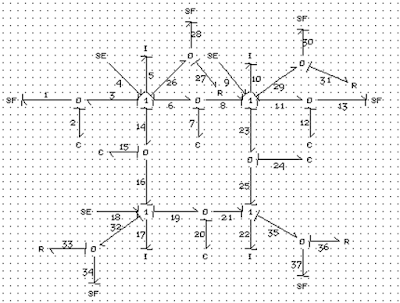Figure 10: Showing the model of the system in CAMPG software.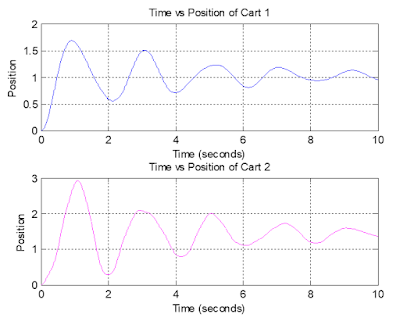Figure 11: Showing the position of the cart 1 and 2.Figure 12: Showing the position of the cart 3 and 4.

Equilibrium position of the carts:
First way:
In this case, according to the question the simulation is run for longer time and the friction coefficient for each mass is changed to 100.Figure 13: Showing the equilibrium position of the cart 1.Figure 14: Showing the equilibrium position of the cart 1 (magnified).

From figure 14, the equilibrium position of the cart 1 is approximately 1.035 unit.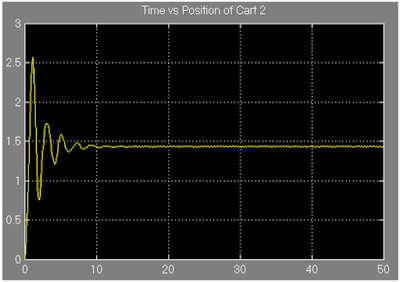Figure 15: Showing the equilibrium position of the cart 2.Figure 16: Showing the equilibrium position of the cart 2 (magnified).

From figure 16, it is observed that the cart 2 settles when its position is around 1.435 unit.Figure 17: Showing the equilibrium position of the cart 3.Figure 18: Showing the equilibrium position of the cart 3 (magnified).

From figure 18, it is found that the approximate equilibrium position of cart 3 is 2.29 unit.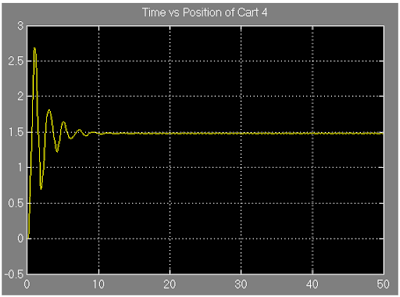Figure 19: Showing the equilibrium position of the cart 4.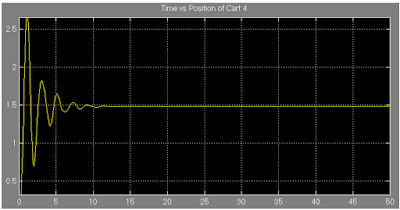Figure 20: Showing the equilibrium position of the cart 4 (magnified).

From figure 19, the approximate equilibrium position is obtained as 1.49 unit.

The following figures 21 and 22 show the equilibrium position of cart 1,2,3, and 4 respectively which further ensure the conformity of the previous simulated results.Figure 21: Showing the equilibrium position of the cart 1 and 2.Figure 22: Showing the equilibrium position of the cart 3 and 4.

Second way:

The concept of steady state is implemented here to find out the equilibrium position of each cart. To do so, the derivative terms are considered zero. The four algebraic equations are obtained:

(1)

(2)
(3)
(4)

Substituting x1 from equation (1),
Substituting the value of x1 and x2 from equation (1) and (2) respectively,
Finally, substituting the value of x2 and x3 from equation (2) and (3) in equation (4);
x4=1.6 unit
Substituting the value of x4 in equation 3;
x3=2.34 unit
Substituting the values of x3 and x4 in equation 2;
x2=1.54 unit
Substituting the value of x2 and x3 inequation 1;
x1=1.05 unit

References:
 D. C. Karnopp, D. L. Margolis and R. C. Rosenberg, ”System Dynamics: Modeling and Simulation of Mechatronic Systems”, fourth edition, John Wiley and Sons, New Jersey, 2006.
 http://www.bondgraph.com/